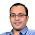## Dec 4, 2011

### Linked List Delete

Source: Asked to me by Ankush Jain (CSE IITB 2011 Alumnus, Morgan Stanley Quant)

Problem:
You are given a pointer to a node (not the tail node) in a singly linked list. Delete that node from the linked list. Write code in C.

Update (December 13, 2011):
Solution posted by Siddhartha in comments! Interesting comment by me in comments!

1.Probably one of the most popular CS Interview questions.

boolean delete(Node* node){
if(node->next==NULL) return false;
else{
node->val=node->next->val;
node->next=node->next->next;
return true;
}
}

2.If playing with the data part of the node is allowed, copying the next node into the current node and then deleting the next node will suffice.

3.Another interesting question on Linked List,which I was asked recently for one of my Job interviews : You are given a linked list(the head pointer). One of the nodes in the linked list points to an earlier node in the list(a loop). Find that 'earlier' node to which this node points to. Time Complexity : O(n)
Space Complexity O(1).

4.In the C code for the linked list, are you allowed access to the data?
If so, copy the data for the next node to this node, and then delete the next node. (by setting the "next" pointer of this node (say n) to n->next->next)

5.If O(n) can't be done, then the only way I can think of is to move next node data to the current node and delete the next node. But you need to assume that there aren't other pointers to these nodes - else you are making some valid pointers (valid in the O(n) soln) invalid and vice versa which may not really be the right thing to do in the context of the program.

6.This comment has been removed by the author.

7.Let the pointer to the node be point.Then the code is as follows:

current=point->next->next;point->info=point->next->info;
point->next=current;

8.Ankush has already given the correct solution.
However, just for technical details sake, we need to free the next node too using free(node->next)
So another possible solution might be(copying the rest of the code from ankush)

boolean delete(Node* node){
if(node->next==NULL) return false;
else{
node->val=node->next->val;
struct node *temp=node->next;
node->next=node->next->next;
free(temp);
return true;
}
}

9.All comments point to the correct solution, but Siddhartha's solution is most apt. Thanks.

One point I would like to make:
If the pointer is the last element of the linked list, then is there no way to delete the last element in O(1).. or is there? In Siddhartha's code, "if(node->next==NULL) return false; " does not delete the node. Thanks

10.Verified my point with smarter people - All agree with me. So, we have O(1) solution provided the node is not the tail. If the node is a tail, we have O(n) algorithm.

Thanks

### Fraction Brainteaser

Source: Sent to me by Gaurav Sinha Problem: Siddhant writes a Maths test and correctly answers 5 out of 6 Arithmetic questions and 20...